Merge Sort

Merge sort is a well-known and widely used sorting method that sorts an array list of integers, characters, or strings using a divide-and-conquer technique. Some reasons to learn this merge sort are listed below.

Algorithm:

· In many sorting algorithms, this is the fastest sorting algorithm. [Time complexity of O(nlogn)]

· A common linked list sorting method with an O(nlog n) time complexity.

· One of the greatest algorithms for learning recursion design and analysis.

· The most effective method for dealing with pointers. To construct a partial solution, both pointers travel in the same direction. We can use the same strategy to solve other issues as well.

How does it work?

1. Return if there is only one entry in the list that has already been sorted.

2. Recursively divide the list into two pieces until it can no longer be divided.

3. Merge the smaller lists into a new list in the order they were created.

Rule of Divide and Conquer:

Consider the task of sorting an array of n numbers from index I to index r. The goal is to solve a sorting issue of size n by solving smaller sub-problems or utilizing the divide and conquer strategy.

Ø Divide:

Divide the n-part sorting problem into two separate and equal subparts.

· Sorting A from 1 to mid is the left subproblem.

· Sorting A from mid +1 or r is the right subproblem.

Ø Conquer:

Both subproblems are solved recursively, and the smaller halves are sorted. We don’t have to worry about finding a solution to this problem because recursion will take care of it.

Ø Combine parts:

The final sorted array is created by combining the two sorted halves. Please answer the sorting problems of size n once we have solved both subproblems of size n/2.

Implementation of Merge Sort:

· Divide part: we call the mid-value Mid= I + (r-I)/2.

·

Here, arr denotes the specified array, beg denotes the array’s first element, and end denotes the array’s last element. The MERGE function is a crucial feature of the merge sort. This function merges two sorted sub-arrays, A[beg…mid] and A[mid+1…end], to create one sorted array A[beg…end]. A[], beg, mid, and end are the MERGE function’s inputs.

· Conquer part: We use the same method with mid + 1 as the left end and recursively sort the right half of size n/2.

· The merge(A, l, mid, r) function is used within the mergeSort() function to merge both smaller sorted halves into a final sorted array.

Base case:

If we find l == r during the recursive calls, the sub-array has only one element left, which can be sorted easily. As a result, recursion will stop here and return. In other words, the smallest version of the sorting issue for which recursion yields the answer directly is the sub-array of size one.

--

--

--

## Understanding Mobx — behind the scenes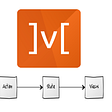## How to Identify, Diagnose, and Fix Memory Leaks in Web Apps## GraphQL — Data access based on users’ roles with express-graphql## Using javascript to create an object in a rails API## Infinite Scroll With React Hooks## Find Callbacks Confusing? They are Nothing But…## How to manipulate the DOM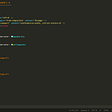## 5 Tips Before Deploying your Angular Apps to Production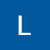## Backtracking Algorithm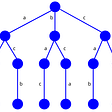## Behavior of the objects.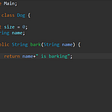## What is a Singly Linked List?